# Solutions, Electrolytes and Nonelectrolytes

An error occurred trying to load this video.

Try refreshing the page, or contact customer support.

Coming up next: Solubility and Solubility Curves

### You're on a roll. Keep up the good work!

Replay
Your next lesson will play in 10 seconds
• 0:01 Solutions
• 2:52 Calculating Molarity,…
• 6:04 Electrolytes and…
• 7:20 Ionic and Covalent Substances
• 9:02 Lesson Summary
Save Save

Want to watch this again later?

Timeline
Autoplay
Autoplay
Speed Speed

#### Recommended Lessons and Courses for You

Lesson Transcript
Instructor: Elizabeth (Nikki) Wyman

Nikki has a master's degree in teaching chemistry and has taught high school chemistry, biology and astronomy.

Learn what a solution is and how it is formed. Learn how to express the concentration of a solution in terms of molarity, molality and mass percent. Discover the differences between an electrolyte and a nonelectrolyte.

## Solutions

Most things in your daily life are mixtures. Milk is a mixture. Shampoo is a mixture. Air is a mixture. A mixture is two or more substances that are mixed but are not chemically combined.

There are two different kinds of mixtures: heterogeneous and homogenous. In a heterogeneous mixture like salsa or chicken noodle soup, the different substances are distinguishable from one another. In homogenous mixtures, the different substances inside are indistinguishable from one another. The physical properties of a homogenous mixture like air or Gatorade are uniform.

Solutions are homogenous mixtures made of a solute and a solvent. Solutes are substances that get dissolved. Solvents are the substances that do the dissolving.

Solutions can be made of any two phases of matter. Solutions may be made up of gases dissolved in gases, or solids dissolved in liquids, or liquids dissolved in liquids. Most of the time, solutions are made up of a solid solute and liquid solvent. Water is often referred to as the universal solvent because it is capable of dissolving so many different substances. In this lesson, we will be looking at examples of solutions made of solid solutes and water as the solvent.

One of the most important qualities of a solution is its concentration. A solution isn't chemically combined, so it can have different amounts of solute dissolved in the solvent. More solute per solvent means a more concentrated solution. Similarly, less solute per solvent means a less concentrated solution. The concentration of a solution is most frequently described in terms of molarity. Molarity is the number of moles of a solute per liter of solution. It has the units M or mol/L.

Concentration of a solution may also be described in terms of molality. Molality is the mass of the solute per kilogram of solvent.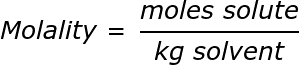The unit for molality is an italicized, lowercase m. Molality is useful when performing calculations involving the boiling or freezing points of solutions.

Lastly, solution concentration can also be described by mass percent, which is the percent by mass of the solute in the solution. Mass percent can be calculated by dividing the mass of the solute by mass of solution and multiplying the result by 100%. Mass percent is often used in analysis of solutions, like water quality reports.

## Calculating Molarity, Molality and Mass Percent

Imagine you work for a chemical supply company, and for every solution you make, you must give a report on concentration in terms of molarity, molality and mass percent.

Today, you have to dissolve 85.58 g of sucrose (table sugar) in 1.5 L of water. The molar mass of sucrose is 342.3 g per mole.

The first task on our list is to calculate molarity for this solution using the molarity equation: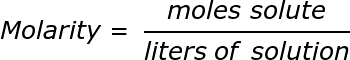We have liters of solution given to us but must calculate number of moles given both the mass of sucrose and the molar mass of sucrose.

To find moles of sucrose, we divide the given mass of sucrose by the molar mass of sucrose: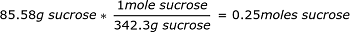Now, we divide our number of moles solute by our liters of solution to find molarity: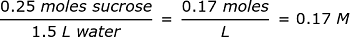Our next task is to find molality using our molality equation:We know that we have 0.25 moles of solute - we just figured out that in the previous problem - and 1.5 liters of solvent. We must convert liters solvent to kilogram solvent. The density of water is 1 kg/L, meaning that the mass of one liter of water is equal to one kilogram. We have 1.5 L of water, equal to 1.5 kg of water.

Now we divide our number of moles solute by kilograms of solvent to find molality: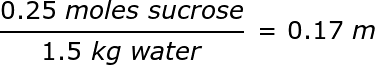To determine the mass percent of the same solution, we would use the following equation: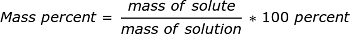For this, it is critical to have the same units for mass for both the solute and the solution. I'm going to use grams.

We have 85.58 grams of sucrose and 1.5 kg of water. There are 1,000 g in 1 kg, so we have 1500 g of water. Notice that we add the mass of the solvent to the mass of the solute to find the total mass of the solution. Now we plug this information into our equation and solve.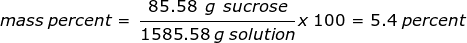We've finished our report and can move on to the next item.

## Electrolytes and Nonelectrolytes

The similarity between the term 'electrolyte' and 'electricity' is no coincidence! In the 1880s, Swedish chemist Svante Arrhenius recognized that some solutions could conduct electricity. He noted that the number of dissolved ions in solution is directly related to the strength of the solution's electrical potential. Compounds that dissolve by breaking into ions and conduct electricity in solution are known as electrolytes. Most soluble salts, acids and bases are electrolytes.

Compounds that dissolve in water but do not conduct electricity are known as nonelectrolytes. Nonelectrolytes are often covalently bonded compounds like sugars or alcohols.

To unlock this lesson you must be a Study.com Member.

### Register to view this lesson

Are you a student or a teacher?

#### See for yourself why 30 million people use Study.com

##### Become a Study.com member and start learning now.
Back
What teachers are saying about Study.com

### Earning College Credit

Did you know… We have over 200 college courses that prepare you to earn credit by exam that is accepted by over 1,500 colleges and universities. You can test out of the first two years of college and save thousands off your degree. Anyone can earn credit-by-exam regardless of age or education level.